# Set Game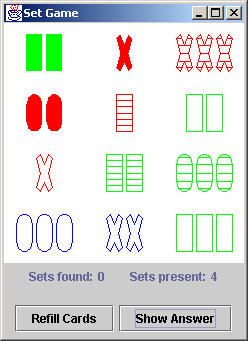The game is played by finding sets of three cards that are all the same, or all different, across four independent "facets" or "dimensions". These dimensions are: number, shape, color, and fill. Each of these dimensions has three possible values. Number has: one, two, or three; color has: red, green, or blue; shape has: rectangle, X, or O; and fill has: open, striped, or solid.

Here are three cards that demonstrate all twelve values: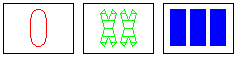Let's look at some examples. The following three cards are all the same in: number, color, and shape. They are all different in: fill. Because each of the four dimensions are all the same, or, all different, these three cards form a set.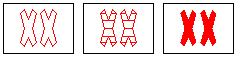The next three cards are the same in: number, and shape. They are different in: color, and fill. Each of the four dimensions is either entirely homogeneous or entirely different; therefore, this is a set.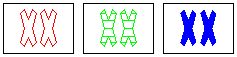What about the three cards below? We have: same number, same shape, different colors, two open fills, and one striped fill. In order for this to be a set, the fills have to be all the same, or all different. So either the two green Xs have to be open, or, one of the other cards has to be solid.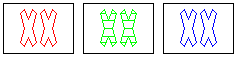The next three cards are the same in shape; and different in color, number, and fill. This is a set.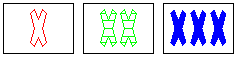Below, we have the same shape and number, different fill, two reds, and one green not a set. To make it a set, one of the red cards needs to be blue, or the green card needs to be red.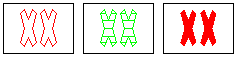Finally, here is a set where all 4 dimensions are different.Can you find the four sets in the first window of this chapter? The answers are listed in the window displayed in the Iteration 9 section.

At their Web site, the people who invented SET? suggest it is an excellent vehicle for exercising both halfs of your brain. Because the game involves objective rules, players must exercise "left brain" logical, sequential problem solving. However, to find the sets, players must examine the two dimensional arrangement of cards and locate patterns with "right brain" intuitive, spatial perception.

"To effectively employ creative thinking requires use of both left and right sides of your brain. Both right brain thinking skills, and whole brain thinking receive little attention in school. They remain underdeveloped as we go through life because only a few occupations such as a football quarterback, pilot, or artist require them. However, everyone will gain by developing them. Every time you find a set you are using your whole brain and increasing your potential to be creative."

The total number of cards in a SET deck is 81. This is the number of permutations of three variables that each take on three possible values [(3 numbers) * (3 colors) * (3 shapes) * (3 fills) = 81].

Twelve cards are initially displayed on the table. As players identify and pick up sets, the holes are filled by dealing replacement cards. The game is over when all the cards have been dealt, and no more sets can be formed. The maximum number of sets is 27. Because each individual card can participate in quite a few set, it is possible to find yourself at the end of the game with several cards that cannot be matched.

### Set - and the software professional

I think programmer's are pathologically left-brain by nature: an algorithm for everything and everything in its algorithm, schema, flowchart, state machine, collaboration diagram, etc.

Only with conscious effort, do we aspire to, and mature in practicing, the art of abstraction. Grady Booch has suggested, "Only about 20-30% of software developers are probably really good at OO abstraction. That doesn't mean the remaining 70-80% are inadequate. Rather, this is a recognition of the fact that some people are better than others at looking at the world and discovering or inventing abstractions of reality."

The practice of abstraction can be strongly linked to the pattern recognition and spatial perception of right-brain thinking skills. The practice of programming (tied as it has been to sequence, selection, and iteration) is routinely prejudiced towards the logical orientation and linear perception of left-brain thinking skills. To succeed in our domains of ever expanding complexity, software architects and practitioners must integrate and leverage their "whole" brain.

Years ago, I heard a definition for innovation - the ability to see what everyone else has seen and think what no one else has thought. So much of this art referred to as "seeing" is really the ability to discern patterns that have heretofore gone unharvested. The SET game is an excellent vehicle for bolstering one's powers of discovery. Software design is a prime beneficiary of expanded powers of discernment.

To the degree that the act of software design remains a discipline characterized by creativity and craftsmanship, there is no substitute for multi-disciplinary powers of perception. Whether these additional dimensions of insight come from mathematical card games, or the competing world views of software design paradigms; every tool we can bring to bear on the systems we build will result in a whole that is greater than the sum of its parts.

Learn and leverage as many paradigms as possible.

### The project

Start by building basic drawing functionality. Then introduce user interface and control functions. Finally, design and build the model that supports the business logic of the game.

The nine iterations are:

1. Draw 12 blank cards
2. Draw 9 cards - number, shape
3. Draw 9 cards - number, shape, color, fill
4. Encode the 4 dimensions and represent 81 cards
5. Draw 81 cards
6. Select, hilite, and remove 3 cards at a time
7. Shuffle cards, build entire UI, count the number of sets found, refill holes
8. Design the model, validate each set selected
9. Compute all sets present, display the list of sets present
Iteration 3 is an interesting exercise in, I can name that song in 5 notes, Bob. How can you use indirection to draw one, two, three, red, green, blue, rectangle, X, O, open, striped, solid, and 12 cards in a compact function or two.?

Iteration 4 is a remarkable puzzle where insight and data structure will make all the difference. Once weve laid the foundation with an interesting representation, iteration 5 is easy.

How can the validity of a set be evaluated? Iteration 8 is another significant puzzle, and well discover an algorithm that can be implemented in nine lines of code.

### Iteration 1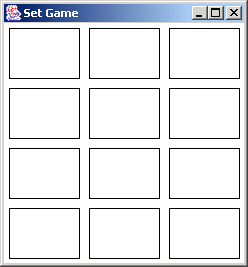To get started, lets limit ourselves to drawing the outline of 12 cards. Use the following dimensions to get yourself started.
```// card width and height
private int CW = 70, CH = 50;
// the left edge for each column
private int[] cardXs = { 5, 85, 165 };
// the top edge for each row
private int[] cardYs = { 5, 65, 125, 185 };
...
setSize( 240, 240 );  // canvas width and height
```
We've previously discussed the practice of using symbolic constants to represent values that are likely to change.
Hard-wiring values produces weak spots in a design that are a lightning rod for change.
Instructions and issues:
• Copy the JFrame code from the previous chapter
• Use a Canvas component and the drawRect() method of the AWT Graphics class
• Make sure the application redraws itself when expose events occur

### Iteration 2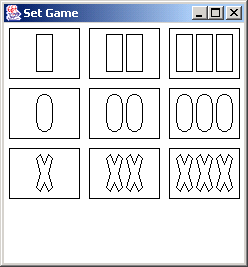The AWT Graphics class allows shapes to be drawn using the method drawPolygon(polygonXs,polygonYs,numberOfPoints). The outline of the three types of shapes are given below. The first row draws a rectangle, the second row an O, and the final row an X.

The X offset for each shape in one, two, and three-shape cards are also provided.

```// shapes: rectangle, O, X
private int[][] shapeXs = { { 0, 16, 16, 0, 0 },
{ 0, 2, 6, 10, 14, 16, 16, 14, 10, 6, 2, 0, 0 },
{ 0, 4, 8, 12, 16, 12, 16, 12,  8, 4, 0, 4, 0 } };
private int[][] shapeYs = { { 0, 0, 37, 37, 0 },
{ 10, 3,  0, 0, 3, 10, 27, 34, 37, 37, 34, 27, 10 },
{ 4, 0, 10, 0, 4, 19, 33, 37, 28, 37, 33, 19, 4 } };
// numbers: one, two, three
private int[][] one23Xs = { { 27 }, { 17,37 }, { 7,27,47 } };
// Y margin for the top of each shape
private int SY = 6;
...
g.drawPolygon( polygonXs, polygonYs, numberOfPoints );
```
In this iteration, develop the code for drawing two of our four dimensions: shape, and number. You will need to add together contributions from several data structures to draw each shape within each card.

Instructions and issues:

• Draw nine cards only

### Iteration 3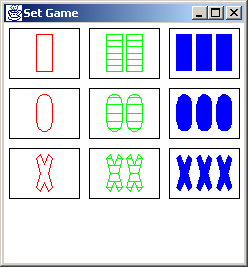Add the last two dimensions (color and fill) in this iteration. Use the drawPolygon(polygonXs,polygonYs,numberOfPoints) method for solid fill. For striped fill, use the relative coordinate arrays below.
```private int[][] shapeXs = {
{ 0, 16, 16, 0, 16, 16, 0, 16, 16, 0, 16, 16,
0, 16, 16, 0, 16, 16, 0, 0 },
{ 0, 2, 6, 10, 14, 2, 14, 16, 0, 16, 16, 0,
16, 16, 0, 16, 14, 2, 14, 10, 6, 2, 0, 0 },
{ 0, 4, 8, 2, 14, 8, 12, 16, 11, 16, 12, 4,
12, 16, 11, 16, 12,  8,  2, 14, 8, 4, 0, 5,
0, 4, 0, 5, 0 } };
private int[][] shapeYs = {
{ 0, 0, 6, 6, 6, 12, 12, 12, 19, 19, 19, 25,
25, 25, 31, 31, 31, 37, 37, 0 },
{ 10, 3,  0, 0, 3, 3, 3, 10, 10, 10, 19, 19, 19,
27, 27, 27, 34, 34, 34, 37, 37, 34, 27, 10 },
{ 4, 0, 11, 11, 11, 11, 0, 4, 4, 4, 19, 19, 19,
33, 33, 33, 37, 27, 27, 27, 27, 37, 33, 33,
33, 19, 4, 4, 4 } };
```
The fill dimension seems to be usually involved. The open fill and striped fill use two different arrays of relative X,Y values and the drawPolygon() instruction; while the solid fill uses the fillPolygon() instruction and the same relative X,Y arrays as the open fill.

Instructions and issues: How do you want to handle the two different sets of relative X,Y values? Do you need to keep them separate and use an if-else test to access the correct set; or, can you merge the two sets into one and compute the appropriate offset?

### Iteration 4

Encode the 4 dimensions and represent 81 cards

All permutations of four 3-value variables

 0000     1000 2000 0100 1100 2100 0200 1200 2200 0010     1010 2010 0110 1110 2110 0210 1210 2210 0020     1020 2020 0120 1120 2120 0220 1220 2220 0001     1001 2001 0101 1101 2101 0201 1201 2201 0011     1011 2011 0111 1111 2111 0211 1211 2211 0021     1021 2021 0121 1121 2121 0221 1221 2221 0002     1002 2002 0102 1102 2102 0202 1202 2202 0012     1012 2012 0112 1112 2112 0212 1212 2212 0022     1022 2022 0122 1122 2122 0222 1222 2222
Instructions and issues:

### Iteration 5

Draw 81 cards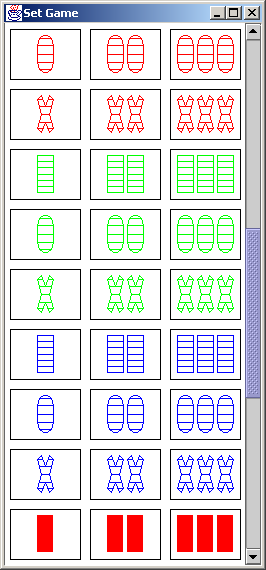Instructions and issues:

### Iteration 6

Select, hilite, and remove 3 cards at a time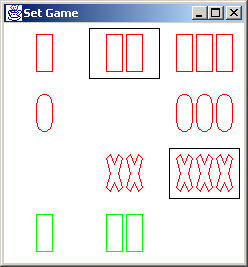Instructions and issues:

### Iteration 7

Shuffle cards, build entire UI, count the number of sets found, refill holes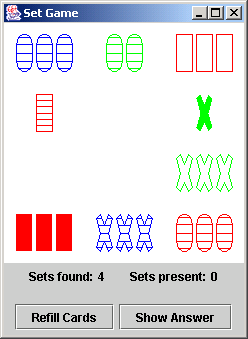Instructions and issues:

### Iteration 8

Design the model, validate each set selected

```	0	0	1	1	2	2	0	0	1	2
0	1	1	2	2	0	1	0	1	2
1	1	2	2	0	0	2	0	1	2
set?	no	no	no	no	no	no	yes	yes	yes	yes
sum	1	2	4	5	4	2	3	0	3	6

dim1	dim2	dim3	dim4
card1	0	2	1	0
card2	0	2	1	1
card3	0	2	1	2
sum	0	6	3	3
set?	yes

dim1	dim2	dim3	dim4
card1	0	1	2	2
card2	0	1	2	2
card3	1	2	0	1
sum	1	4	4	5
set?	no
```
Instructions and issues:

### Iteration 9

Compute all sets present, display the list of sets present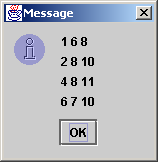Instructions and issues:

### Iteration 1 - implementation

We need to draw four rows and three columns. We could use an inner loop for the columns, and an outer loop for the rows.
```public void paint( Graphics g ) {
for (int i=0; i < cardYs.length; i++)
for (int j=0; j < cardXs.length; j++)
g.drawRect( cardXs[j], cardYs[i], CW, CH );
}
```
Or - we could remove one level of bookkeeping, and use the modulus operator and integer division to achieve the same end.
```public void paint( Graphics g ) {
for (int i=0; i < 12; i++)
g.drawRect( cardXs[i%3], cardYs[i/3], CW, CH );
}
```
The "i%3" expression cycles through the values 0, 1, and 2. That is exactly what the inner loop did in the previous implementation. This could be described as a "saw-tooth function". Each time the modulus operator jumps back to 0, the integer division expression steps to its next level. That replaces the role of the outer loop.
Integer division is useful as a "stair-step function". The modulus operator yields a "saw-tooth function".
Listing 8.1

```import java.awt.*;
import javax.swing.*;

public class SetGame extends Canvas {
private int   CW = 70, CH = 50;
private int[] cardXs = { 5, 85, 165 };
private int[] cardYs = { 5, 65, 125, 185 };

public static void main( String[] args ) {
new SetGame();
}

public SetGame() {
JFrame frame = new JFrame( "Set Game" );
setSize( 240, 240 );
setBackground( Color.white );
frame.setDefaultCloseOperation( JFrame.EXIT_ON_CLOSE );
frame.pack();
frame.setVisible( true );
}

public void paint( Graphics g ) {
for (int i=0; i < 12; i++)
g.drawRect( cardXs[i%3], cardYs[i/3], CW, CH );
} }
```

### Iteration 2 - implementation

We could think of this problem in terms of applying several "overlays". At the highest level there is the position of each card. Then there is the position of each shape within a card. And finally, the X and Y arrays of each individual polygon. A top level loop is needed to iterate through the nine cards. A lower level loop can iterate through the number of shapes for each card. A third loop can apply the contribution from the other two loops to the most finest-grain component of each individual drawing instruction the X and Y vectors for each shape type. If we encapsulate the third loop in a function, then the return value of the function can report how many elements have been populated in the array parameters passed to the function. Notice that the modulus operator (i.e. saw-tooth function) is being used to visit all elements of the one23Xs array for each row; and the integer division operator (i.e. stair-step function) is used to hold the shape choice constant for each row.
```private int[] polygonXs = new int, polygonYs = new int;
...
for (int i=0; i < 9; i++) {
for (int j=0, length; j < one23Xs[i%3].length; j++) {
length = computeShapeXYs( i/3, cardXs[i%3] + one23Xs[i%3][j],
cardYs[i/3] + SY );
g.drawPolygon( polygonXs, polygonYs, length );
} }
```
The computeShapeXYs() function is oblivious to all the decisions being made externally. Its sole responsibility is to iterate through the designated shapes relative positions, and compute absolute positions.
```private int computeShapeXYs( int i, int x, int y ) {
for (int j=0; j < shapeXs[i].length; j++) {
polygonXs[j] = shapeXs[i][j] + x;
polygonYs[j] = shapeYs[i][j] + y;
}
return shapeXs[i].length;
}
```
The notion of "overlays" put forth at the beginning of this section reminds me of physiology textbooks that represent each sub-system of the human body on its own clear plastic page. The skeletal system, the circulatory system, the respiratory system, the digestive system, and the musculature can be studied in isolation – or – they can be overlayed and examined in concert. In software, this kind of approach has gone by many names: divide and conquer, separation of concerns, layers of abstraction, etc. We used it in this iteration to: decompose the complexity of drawing shapes on cards, and wield remarkable leverage with very little code.
Perspective is everything – separate concerns into "horizontal" layers, and at the last minute overlay them to produce a "vertical" solution.

Listing 8.2

```import java.awt.*;
import javax.swing.*;

public class SetGame extends Canvas {
private int   CW = 70, CH = 50, SY = 6;
private int[] cardXs = { 5, 85, 165 };
private int[] cardYs = { 5, 65, 125, 185 };
private int[][] shapeXs = { { 0, 16, 16, 0, 0 },
{ 0, 2, 6, 10, 14, 16, 16, 14, 10, 6, 2, 0, 0 },
{ 0, 4, 8, 12, 16, 12, 16, 12,  8, 4, 0, 4, 0 } };
private int[][] shapeYs = { { 0, 0, 37, 37, 0 },
{ 10, 3,  0, 0, 3, 10, 27, 34, 37, 37, 34, 27, 10 },
{ 4, 0, 10, 0, 4, 19, 33, 37, 28, 37, 33, 19, 4 } };
private int[][] one23Xs = { { 27 }, { 17,37 }, { 7,27,47 } };
private int[]   polygonXs = new int, polygonYs = new int;

public static void main( String[] args ) { ... }
public SetGame() { ... }

public void paint( Graphics g ) {
for (int i=0; i < 9; i++) {
g.drawRect( cardXs[i%3], cardYs[i/3], CW, CH );
for (int j=0, length; j < one23Xs[i%3].length; j++) {
length = computeShapeXYs( i/3, cardXs[i%3] + one23Xs[i%3][j],
cardYs[i/3] + SY );
g.drawPolygon( polygonXs, polygonYs, length );
} } }

private int computeShapeXYs( int i, int x, int y ) {
for (int j=0; j < shapeXs[i].length; j++) {
polygonXs[j] = shapeXs[i][j] + x;
polygonYs[j] = shapeYs[i][j] + y;
}
return shapeXs[i].length;
} }
```

### Iteration 3 - implementation

Drawing the new dimension of color should be easy. Like we did with the dimensions of number and fill, lets create yet another array to hold the legal color values. If we wanted to hold the color constant for each row (like we are doing for the dimension of shape), then we would need the expression "i/3". But, the assignment called for varying color like we are already varying the dimension of number, so, the expression "i%3" is appropriate.
```private Color[] colors = { Color.red, Color.green, Color.blue };
...
for (int i=0; i < 9; i++) {
for (int j=0, length; j < one23Xs[i%3].length; j++) {
length = computeShapeXYs( i/3, cardXs[i%3] + one23Xs[i%3][j],
cardYs[i/3] + SY );
g.setColor( colors[i%3] );
g.drawPolygon( polygonXs, polygonYs, length );
}
```
For the dimension of fill, we could choose to create brand new data structures like the following.
```private int[][] shapeXs = { ... };
private int[][] shapeYs = { ... };
private int[][] stripeXs = {
{ 0, 16, 16, 0, 16, 16, 0, 16, 16, 0, 16, 16, 0, 16, 16, 0, 16, 16, 0, 0 }, ... };
private int[][] stripeYs = {
{ 0, 0, 6, 6, 6, 12, 12, 12, 19, 19, 19, 25, 25, 25, 31, 31, 31, 37, 37, 0 }, ... };
```
The fill dimension needs to cycle in step with the number and color dimensions, so the expression "i%3" will be necessary to compute array indices. When "i%3" is equal to 0, the fill should be open. When "i%3" is 1, the fill is striped. And "i%3" equal to 2 means a fill of solid. Since the fill affects how the polygon Xs and Ys are computed, the method computeShapeXYs() will need to change.
```private int computeShapeXYs( int i, int x, int y ) {
if (i%3 == 1) {
for (int j=0; j < stripeXs[i].length; j++) {
polygonXs[j] = stripeXs[i][j] + x;
polygonYs[j] = stripeYs[i][j] + y;
}
return stripeXs[i].length;
} else {
for (int j=0; j < shapeXs[i].length; j++) {
polygonXs[j] = shapeXs[i][j] + x;
polygonYs[j] = shapeYs[i][j] + y;
}
return shapeXs[i].length;
} }
```
asd
```private int[][] shapeXs = { openRect, openO, openX, stripedRect, stripedO, stripedX };

public void paint( Graphics g ) {
for (int i=0; ... ) {
...
for (int j=0, ... ) {
length = computeShapeXYs( i, ... );
...
} } }

private int computeShapeXYs( int i, int x, int y ) {
int shapeIndex = i/3 + (3 * (i%3 == 1 ? 1 : 0));
for (int j=0; j < shapeXs[shapeIndex].length; j++) {
polygonXs[j] = shapeXs[shapeIndex][j] + x;
polygonYs[j] = shapeYs[shapeIndex][j] + y;
}
return shapeXs[shapeIndex].length;
}
```
asd
```private int[][] shapeXs = { openRect, stripedRect, openO, stripedO, openX, stripedX };

private int computeShapeXYs( int i, int x, int y ) {
int shapeIndex = i/3 * 2 + (i%3 == 1 ? 1 : 0);
...
```
The method paint() needs to decide whether to invoke drawPolygon() or fillPolygon().
```public void paint( Graphics g ) {
...
g.setColor( colors[i%3] );
if (i%3 == 2)
g.fillPolygon( polygonXs, polygonYs, length );
else
g.drawPolygon( polygonXs, polygonYs, length );
} }
```
Listing 8.3

```import java.awt.*;
import javax.swing.*;

public class SetGame extends Canvas {
private int   CW = 70, CH = 50, SY = 6;
private int[] cardXs = { 5, 85, 165 };
private int[] cardYs = { 5, 65, 125, 185 };
private int[][] shapeXs = { { 0, 16, 16, 0, 0 },
{ 0, 16, 16, 0, 16, 16, 0, 16, 16, 0, 16, 16, 0, 16, 16, 0, 16, 16, 0, 0 },
{ 0, 2, 6, 10, 14, 16, 16, 14, 10, 6, 2, 0, 0 },
{ 0, 2, 6, 10, 14, 2, 14, 16, 0, 16, 16, 0, 16, 16, 0, 16, 14, 2, 14, 10,
6, 2, 0, 0 },
{ 0, 4, 8, 12, 16, 12, 16, 12,  8, 4, 0, 4, 0 },
{ 0, 4, 8,  2, 14, 8, 12, 16, 11, 16, 12, 4, 12, 16, 11, 16, 12,  8,  2, 14,
8, 4, 0,  5,  0,  4, 0, 5, 0 } };
private int[][] shapeYs = { { 0, 0, 37, 37, 0 },
{ 0, 0, 6, 6, 6, 12, 12, 12, 19, 19, 19, 25, 25, 25, 31, 31, 31, 37, 37, 0 },
{ 10, 3,  0, 0, 3, 10, 27, 34, 37, 37, 34, 27, 10 },
{ 10, 3,  0, 0, 3, 3, 3, 10, 10, 10, 19, 19, 19, 27, 27, 27, 34, 34, 34, 37,
37, 34, 27, 10 },
{ 4, 0, 10, 0, 4, 19, 33, 37, 28, 37, 33, 19, 4 },
{ 4, 0, 11, 11, 11, 11,  0,  4,  4,  4, 19, 19, 19, 33, 33, 33, 37, 27, 27,
27, 27, 37, 33, 33, 33, 19, 4, 4, 4 } };
private int[][] one23Xs = { { 27 }, { 17,37 }, { 7,27,47 } };
private int[]   polygonXs = new int, polygonYs = new int;
private Color[] colors = { Color.red, Color.green, Color.blue };

public static void main( String[] args ) { ... }
public SetGame() { ... }

public void paint( Graphics g ) {
for (int i=0; i < 9; i++) {
g.setColor( Color.black );
g.drawRect( cardXs[i%3], cardYs[i/3], CW, CH );
for (int j=0, length; j < one23Xs[i%3].length; j++) {
length = computeShapeXYs( i, cardXs[i%3] + one23Xs[i%3][j],
cardYs[i/3] + SY );
g.setColor( colors[i%3] );
if (i%3 == 2)
g.fillPolygon( polygonXs, polygonYs, length );
else
g.drawPolygon( polygonXs, polygonYs, length );
} } }

private int computeShapeXYs( int i, int x, int y ) {
int shapeIndex = i/3 * 2 + (i%3 == 1 ? 1 : 0);
for (int j=0; j < shapeXs[shapeIndex].length; j++) {
polygonXs[j] = shapeXs[shapeIndex][j] + x;
polygonYs[j] = shapeYs[shapeIndex][j] + y;
}
return shapeXs[shapeIndex].length;
} }
```

### Iteration 4 - implementation

Encode the 4 dimensions and represent 81 cards

Listing 8.4

```as
```

### Iteration 5 - implementation

Draw 81 cards

Listing 8.5

```as
```

### Iteration 6 - implementation

Select, hilite, and remove 3 cards at a time

Listing 8.6

```as
```

### Iteration 7 - implementation

Shuffle cards, build entire UI, count the number of sets found, refill holes

Listing 8.7

```as
```

### Iteration 8 - implementation

Design the model, validate each set selected

The problem at hand is: how to decide (or compute) whether any arbitrary combination of three Card objects (i.e. three entries from table 2) represent a set. One approach could be to loop through each of the three dimensions, and examine the corresponding digits for each dimension on all three Cards.

To this end, let's enumerate all combinations of the digits 0, 1, and 2 (leaving out all permutations that represent different orderings of the same data). The result would be table 3. The "012" column represents a "different" set; and the "000", "111", "222" columns are "same" sets. The first six columns of digits do not represent sets.

On a hunch, let's try adding each column. All the columns that represent sets are found to be evenly divisible by three. All the columns that don't represent sets are not. That is a remarkable property that significantly simplifies the evaluation of "set-hood".

Given this special "modulus" property, the implementation of the isValid() method is easy. An outer loop can iterate over the number of facets in our domain, and an inner loop can iterate over the number of Cards in a set. If the sum of any of the facets is not evenly divisible by three, then the current combination cannot be a set.

Listing 8.8

### Iteration 9 - implementation

Compute all sets present, display the list of sets present

Listing 8.9